# Selecting rows in pandas DataFrame based on conditions

• Difficulty Level : Medium
• Last Updated : 10 Jun, 2021

Let’s see how to Select rows based on some conditions in Pandas DataFrame.

### Selecting rows based on particular column value using `'>', '=', '=', '<=', '!=' `operator.

Attention geek! Strengthen your foundations with the Python Programming Foundation Course and learn the basics.

To begin with, your interview preparations Enhance your Data Structures concepts with the Python DS Course. And to begin with your Machine Learning Journey, join the Machine Learning - Basic Level Course

Code #1 : Selecting all the rows from the given dataframe in which ‘Percentage’ is greater than 80 using basic method.

 `# importing pandas``import` `pandas as pd`` ` `record ``=` `{`` ` ` ``'Name'``: [``'Ankit'``, ``'Amit'``, ``'Aishwarya'``, ``'Priyanka'``, ``'Priya'``, ``'Shaurya'` `],`` ``'Age'``: [``21``, ``19``, ``20``, ``18``, ``17``, ``21``],`` ``'Stream'``: [``'Math'``, ``'Commerce'``, ``'Science'``, ``'Math'``, ``'Math'``, ``'Science'``],`` ``'Percentage'``: [``88``, ``92``, ``95``, ``70``, ``65``, ``78``] }`` ` `# create a dataframe``dataframe ``=` `pd.DataFrame(record, columns ``=` `[``'Name'``, ``'Age'``, ``'Stream'``, ``'Percentage'``])`` ` `print``(``"Given Dataframe :\n"``, dataframe) `` ` `# selecting rows based on condition``rslt_df ``=` `dataframe[dataframe[``'Percentage'``] > ``80``]`` ` `print``(``'\nResult dataframe :\n'``, rslt_df)`

Output :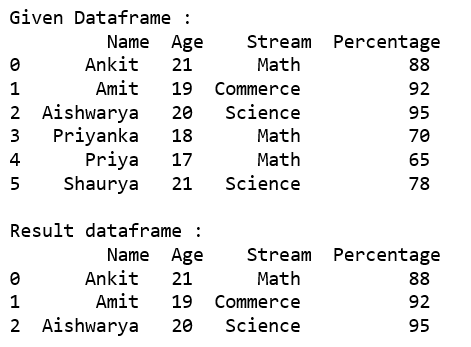Code #2 : Selecting all the rows from the given dataframe in which ‘Percentage’ is greater than 80 using `loc[]`.

 `# importing pandas``import` `pandas as pd`` ` `record ``=` `{``  ``'Name'``: [``'Ankit'``, ``'Amit'``, ``'Aishwarya'``, ``'Priyanka'``, ``'Priya'``, ``'Shaurya'` `],``  ``'Age'``: [``21``, ``19``, ``20``, ``18``, ``17``, ``21``],``  ``'Stream'``: [``'Math'``, ``'Commerce'``, ``'Science'``, ``'Math'``, ``'Math'``, ``'Science'``],``  ``'Percentage'``: [``88``, ``92``, ``95``, ``70``, ``65``, ``78``]}`` ` `# create a dataframe``dataframe ``=` `pd.DataFrame(record, columns ``=` `[``'Name'``, ``'Age'``, ``'Stream'``, ``'Percentage'``])`` ` `print``(``"Given Dataframe :\n"``, dataframe) `` ` `# selecting rows based on condition``rslt_df ``=` `dataframe.loc[dataframe[``'Percentage'``] > ``80``]`` ` `print``(``'\nResult dataframe :\n'``, rslt_df)`

Output :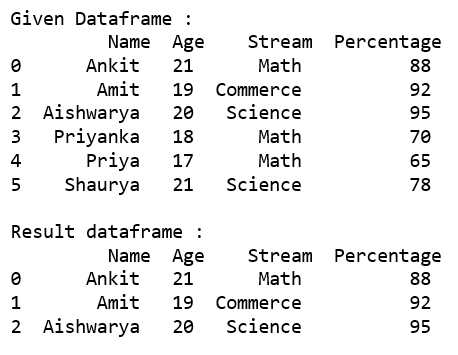Code #3 : Selecting all the rows from the given dataframe in which ‘Percentage’ is not equal to 95 using `loc[]`.

 `# importing pandas``import` `pandas as pd`` ` `record ``=` `{``  ``'Name'``: [``'Ankit'``, ``'Amit'``, ``'Aishwarya'``, ``'Priyanka'``, ``'Priya'``, ``'Shaurya'` `],``  ``'Age'``: [``21``, ``19``, ``20``, ``18``, ``17``, ``21``],``  ``'Stream'``: [``'Math'``, ``'Commerce'``, ``'Science'``, ``'Math'``, ``'Math'``, ``'Science'``],``  ``'Percentage'``: [``88``, ``92``, ``95``, ``70``, ``65``, ``78``]}`` ` `# create a dataframe``dataframe ``=` `pd.DataFrame(record, columns ``=` `[``'Name'``, ``'Age'``, ``'Stream'``, ``'Percentage'``])`` ` `print``(``"Given Dataframe :\n"``, dataframe) `` ` `# selecting rows based on condition``rslt_df ``=` `dataframe.loc[dataframe[``'Percentage'``] !``=` `95``]`` ` `print``(``'\nResult dataframe :\n'``, rslt_df)`

Output :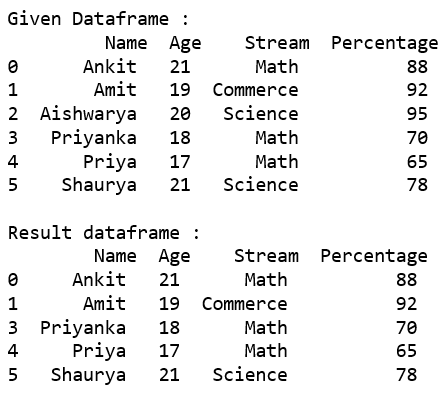### Selecting those rows whose column value is present in the list using `isin()` method of the dataframe.

Code #1 : Selecting all the rows from the given dataframe in which ‘Stream’ is present in the options list using basic method.

 `# importing pandas``import` `pandas as pd`` ` `record ``=` `{``  ``'Name'``: [``'Ankit'``, ``'Amit'``, ``'Aishwarya'``, ``'Priyanka'``, ``'Priya'``, ``'Shaurya'` `],``  ``'Age'``: [``21``, ``19``, ``20``, ``18``, ``17``, ``21``],``  ``'Stream'``: [``'Math'``, ``'Commerce'``, ``'Science'``, ``'Math'``, ``'Math'``, ``'Science'``],``  ``'Percentage'``: [``88``, ``92``, ``95``, ``70``, ``65``, ``78``]}`` ` `# create a dataframe``dataframe ``=` `pd.DataFrame(record, columns ``=` `[``'Name'``, ``'Age'``, ``'Stream'``, ``'Percentage'``])`` ` `print``(``"Given Dataframe :\n"``, dataframe) `` ` `options ``=` `[``'Math'``, ``'Commerce'``]`` ` `# selecting rows based on condition``rslt_df ``=` `dataframe[dataframe[``'Stream'``].isin(options)]`` ` `print``(``'\nResult dataframe :\n'``, rslt_df)`

Output :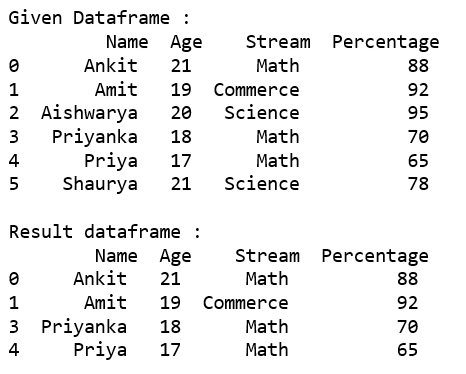Code #2 : Selecting all the rows from the given dataframe in which ‘Stream’ is present in the options list using `loc[]`.

 `# importing pandas``import` `pandas as pd`` ` `record ``=` `{``  ``'Name'``: [``'Ankit'``, ``'Amit'``, ``'Aishwarya'``, ``'Priyanka'``, ``'Priya'``, ``'Shaurya'` `],``  ``'Age'``: [``21``, ``19``, ``20``, ``18``, ``17``, ``21``],``  ``'Stream'``: [``'Math'``, ``'Commerce'``, ``'Science'``, ``'Math'``, ``'Math'``, ``'Science'``],``  ``'Percentage'``: [``88``, ``92``, ``95``, ``70``, ``65``, ``78``]}`` ` `# create a dataframe``dataframe ``=` `pd.DataFrame(record, columns ``=` `[``'Name'``, ``'Age'``, ``'Stream'``, ``'Percentage'``])`` ` `print``(``"Given Dataframe :\n"``, dataframe) `` ` `options ``=` `[``'Math'``, ``'Commerce'``]`` ` `# selecting rows based on condition``rslt_df ``=` `dataframe.loc[dataframe[``'Stream'``].isin(options)]`` ` `print``(``'\nResult dataframe :\n'``, rslt_df)`

Output :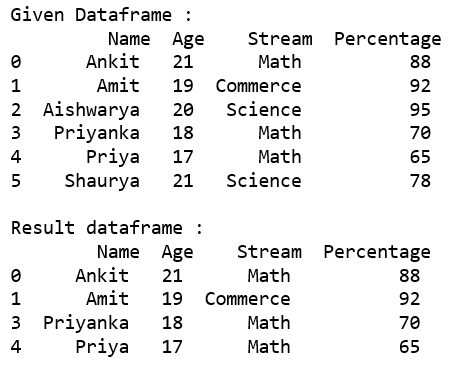Code #3 : Selecting all the rows from the given dataframe in which ‘Stream’ is not present in the options list using `.loc[]`.

 `# importing pandas``import` `pandas as pd`` ` `record ``=` `{``  ``'Name'``: [``'Ankit'``, ``'Amit'``, ``'Aishwarya'``, ``'Priyanka'``, ``'Priya'``, ``'Shaurya'` `],``  ``'Age'``: [``21``, ``19``, ``20``, ``18``, ``17``, ``21``],``  ``'Stream'``: [``'Math'``, ``'Commerce'``, ``'Science'``, ``'Math'``, ``'Math'``, ``'Science'``],``  ``'Percentage'``: [``88``, ``92``, ``95``, ``70``, ``65``, ``78``]}`` ` `# create a dataframe``dataframe ``=` `pd.DataFrame(record, columns ``=` `[``'Name'``, ``'Age'``, ``'Stream'``, ``'Percentage'``])`` ` `print``(``"Given Dataframe :\n"``, dataframe) `` ` `options ``=` `[``'Math'``, ``'Science'``]`` ` `# selecting rows based on condition``rslt_df ``=` `dataframe.loc[~dataframe[``'Stream'``].isin(options)]`` ` `print``(``'\nresult dataframe :\n'``, rslt_df)`

Output :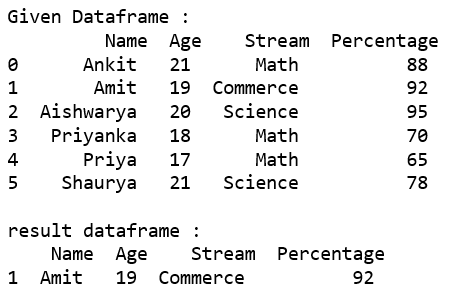### Selecting rows based on multiple column conditions using `'&'` operator.

Code #1 : Selecting all the rows from the given dataframe in which ‘Age’ is equal to 21 and ‘Stream’ is present in the options list using basic method.

 `# importing pandas``import` `pandas as pd`` ` `record ``=` `{``  ``'Name'``: [``'Ankit'``, ``'Amit'``, ``'Aishwarya'``, ``'Priyanka'``, ``'Priya'``, ``'Shaurya'` `],``  ``'Age'``: [``21``, ``19``, ``20``, ``18``, ``17``, ``21``],``  ``'Stream'``: [``'Math'``, ``'Commerce'``, ``'Science'``, ``'Math'``, ``'Math'``, ``'Science'``],``  ``'Percentage'``: [``88``, ``92``, ``95``, ``70``, ``65``, ``78``]}`` ` `# create a dataframe``dataframe ``=` `pd.DataFrame(record, columns ``=` `[``'Name'``, ``'Age'``, ``'Stream'``, ``'Percentage'``])`` ` `print``(``"Given Dataframe :\n"``, dataframe) `` ` `options ``=` `[``'Math'``, ``'Science'``]`` ` `# selecting rows based on condition``rslt_df ``=` `dataframe[(dataframe[``'Age'``] ``=``=` `21``) &``          ``dataframe[``'Stream'``].isin(options)]`` ` `print``(``'\nResult dataframe :\n'``, rslt_df)`

Output :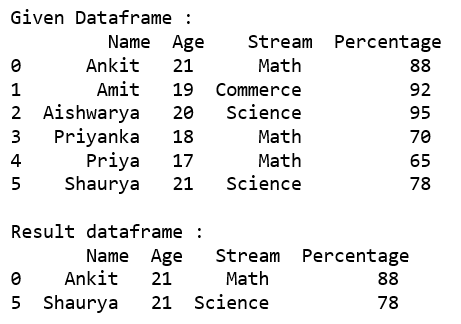Code #2 : Selecting all the rows from the given dataframe in which ‘Age’ is equal to 21 and ‘Stream’ is present in the options list using .loc[].

 `# importing pandas``import` `pandas as pd`` ` `record ``=` `{``  ``'Name'``: [``'Ankit'``, ``'Amit'``, ``'Aishwarya'``, ``'Priyanka'``, ``'Priya'``, ``'Shaurya'` `],``  ``'Age'``: [``21``, ``19``, ``20``, ``18``, ``17``, ``21``],``  ``'Stream'``: [``'Math'``, ``'Commerce'``, ``'Science'``, ``'Math'``, ``'Math'``, ``'Science'``],``  ``'Percentage'``: [``88``, ``92``, ``95``, ``70``, ``65``, ``78``]}`` ` `# create a dataframe``dataframe ``=` `pd.DataFrame(record, columns ``=` `[``'Name'``, ``'Age'``, ``'Stream'``, ``'Percentage'``])`` ` `print``(``"Given Dataframe :\n"``, dataframe) `` ` `options ``=` `[``'Math'``, ``'Science'``]`` ` `# selecting rows based on condition``rslt_df ``=` `dataframe.loc[(dataframe[``'Age'``] ``=``=` `21``) &``              ``dataframe[``'Stream'``].isin(options)]`` ` `print``(``'\nResult dataframe :\n'``, rslt_df)`

Output :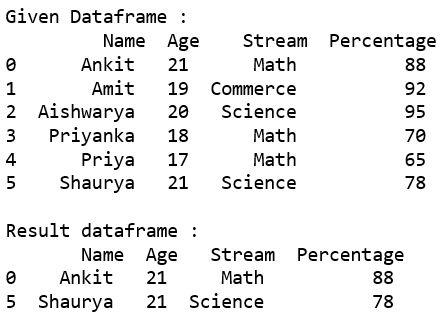My Personal Notes arrow_drop_up